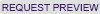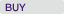Case Detail

Case Summary

Practical Regression: Discrete Dependent Variables

Case Number: 7-112-009, Year Published: 2012

HBS Number: KEL643Key Concepts

Economics, Market Research, Statistical Methods

Abstract

This is the ninth in a series of lecture notes which, if tied together into a textbook, might be entitled “Practical Regression.” The purpose of the notes is to supplement the theoretical content of most statistics texts with practical advice based on nearly three decades of experience of the author, combined with over one hundred years of experience of colleagues who have offered guidance. As the title “Practical Regression” suggests, these notes are a guide to performing regression in practice.
This note returns to the topic of endogeneity, explaining how a predictor variable can be endogenous (and therefore its coefficient can be biased) if causality is in doubt. Through an extended example of the learning curve in medicine, the note introduces the concept of instrumental variables (IV), provides an intuitive explanation for why instruments solve the causality problem, and explains how to estimate IV and two-stage least squares regressions. The note describes statistical tests for the validity of instruments.

Learning Objectives

Students will learn the following:
-Why ambiguities about causality create endogeneity bias
-The intuition behind using IV to solve the endogeneity problem
-How to implement and interpret IV regressions
-Tests for the validity of instruments